Contrasting PROC SSM with Other SAS Procedures

The SSM procedure complements several SAS/ETS procedures and the MIXED procedure in SAS/STAT software (see Chapter 59: The MIXED Procedure in SAS/STAT 12.1 User's Guide,). The statistical models underlying all these procedures can be formulated as state space models; however, in many cases this formulation effort can be considerable. Generally speaking, when a problem can be formulated and satisfactorily solved either by using the SSM procedure or by using one of these other procedures, the other procedures are likely to be more efficient. However, in many instances, the SSM procedure can solve more general problems or offer more detailed analysis, or both. Throughout this discussion, it is assumed that the problem being solved can be modeled as a linear statistical model. In particular, situations that require models such as autoregressive conditional heteroscedasticity (ARCH) models are not considered. The following list provides a more specific comparison of the SSM procedure with different procedures:

• All the SAS/ETS time series analysis procedures (the ARIMA, ESM, UCM, VARMAX, STATESPACE, and PANEL procedures) require time series data and are not applicable to the longitudinal data.

• For univariate time series analysis, the modeling facilities provided by the ARIMA, ESM, and UCM procedures are adequate in most cases. The SSM procedure can handle cases that do not fit neatly into one of these categories.

• For multivariate time series data analysis, you can use the VARMAX procedure for vector ARIMA modeling and the STATESPACE procedure for state space modeling. The capabilities of the SSM procedure are complementary to these procedures. In particular, the predefined multivariate structural models available in the SSM procedure cannot be specified by either of these procedures. In addition, you can formulate a much wider range of multivariate models—for example, models for series with different frequencies, by using the SSM procedure.

• When theside effects are not too complicated (for example, ifis diagonal), the model considered by the MIXED procedure is a special case of the model considered by the SSM procedure. In the case of diagonal, it is easy to see that the state vector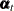is equal to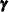, the MIXED random-effects vector, for all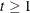(that is,is time invariant). Therefore, the random-effects MIXED model is obtained by setting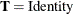,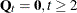,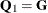(the MIXED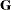matrix), and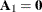.

• For the analysis of cross-sectional data, you can use the PANEL procedure. In this case, the SSM procedure capabilities are complementary. PROC SSM can provide alternate models, REML estimates, richer missing value support, and the estimates of the unobserved components (see the section Getting Started: SSM Procedure and the example Example 27.2 for more information).